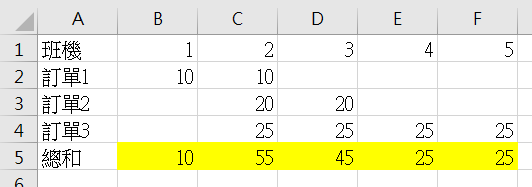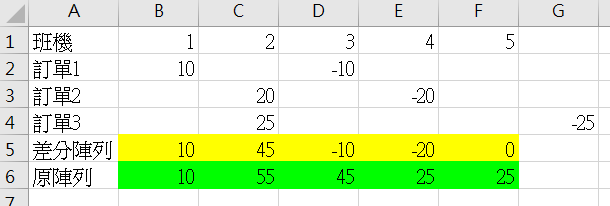#1

## 【小馬的LeetCode練功坊】巧妙解法- 差分陣列 1109. Corporate Flight Bookings

``````Input: bookings = [[1,2,10],[2,3,20],[2,5,25]], n = 5
Output: [10,55,45,25,25]
``````# 解法一: 暴力模擬訂票，超時(時間: O(n^2))

``````class Solution:
def corpFlightBookings(self, bookings: List[List[int]], n: int) -> List[int]:
ans=*n
for b in bookings:
for i in range(b,b+1):
ans[i-1]+=b
return ans
``````

# 觀念- 差分陣列

• `B = A`
• `B[i] = A[i] - A[i - 1]` for i>0

# 解法二: 巧妙，計算差分陣列(時間: O(n))

`Input: bookings = [[1,2,10],[2,3,20],[2,5,25]], n = 5`

<原做法><差分陣列解法>``````class Solution:
def corpFlightBookings(self, bookings , n: int):
diff_arr = *n
for b in bookings:
diff_arr[b-1] += b
if b <n:
diff_arr[b] -= b
result = [] #由差分陣列回推原本的陣列
for e in diff_arr:
result.append(e if not result else result[-1]+e)
return result
``````

``````class Solution {
public:
vector<int> corpFlightBookings(vector<vector<int>>& bookings, int n) {
vector<int> diff_arr(n, 0);
for(auto b: bookings){
diff_arr[b-1] += b;
if(b <n){
diff_arr[b] -= b;
}
}

vector<int> result(n); //由差分陣列回推原本的陣列
result = diff_arr;
for(int i=1;i<n;i++){
result[i]=result[i-1]+diff_arr[i];
}
return result;
}
};
``````

# 類似題型

leetcode上有許多類似題型，